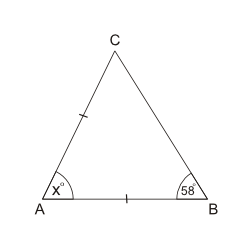We cover every section of the GMAT with in-depth lessons, 5000+ practice questions and realistic practice tests.

## Up to 90+ points GMAT score improvement guarantee

### The best guarantee you’ll find

Our Premium and Ultimate plans guarantee up to 90+ points score increase or your money back.

## Master each section of the test

### Comprehensive GMAT prep

We cover every section of the GMAT with in-depth lessons, 5000+ practice questions and realistic practice tests.

## Schedule-free studying

### Learn on the go

Study whenever and wherever you want with our iOS and Android mobile apps.

# Triangles: IsoscelesIf \$\$AC = AB\$\$, what is the value of \$\$x^\circ\$\$?
Incorrect. [[snippet]] It's a trap! Did you take angle \$\$ABC\$\$ to be equal to angle \$\$BAC\$\$ (\$\$x^\circ\$\$)? Look at the triangle carefully: \$\$AB = AC\$\$ so \$\$\angle ABC = \angle ACB\$\$.
Incorrect. [[snippet]]
Incorrect. [[snippet]]
Correct. [[snippet]] Since \$\$AB = AC\$\$, \$\$\angle ABC = \angle ACB = 58^\circ\$\$. Based on this, > \$\$x^\circ + 58^\circ + 58^\circ = 180^\circ\$\$ > \$\$x^\circ = 180^\circ - 2(58^\circ) = 64^\circ\$\$.
Incorrect. [[snippet]] Did you take angle \$\$ACB\$\$ to be equal to angle \$\$BAC\$\$? Look at the triangle carefully: \$\$AB = AC\$\$ so \$\$\angle ABC = \angle ACB\$\$.
53º
58º
61º
64º
66º